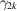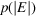International Tables for Crystallography (2006). Vol. B, ch. 2.1, pp. 190-209   | 1 | 2 | https://doi.org/10.1107/97809553602060000554

Contents

• 2.1. Statistical properties of the weighted reciprocal lattice  (pp. 190-209)
• 2.1.1. Introduction  (p. 190) | html | pdf |
• 2.1.2. The average intensity of general reflections  (pp. 190-191) | html | pdf |
• 2.1.2.1. Mathematical background  (pp. 190-191) | html | pdf |
• 2.1.2.2. Physical background  (p. 191) | html | pdf |
• 2.1.2.3. An approximation for organic compounds  (p. 191) | html | pdf |
• 2.1.2.4. Effect of centring  (p. 191) | html | pdf |
• 2.1.3. The average intensity of zones and rows  (pp. 191-192) | html | pdf |
• 2.1.3.1. Symmetry elements producing systematic absences  (pp. 191-192) | html | pdf |
• 2.1.3.2. Symmetry elements not producing systematic absences  (p. 192) | html | pdf |
• 2.1.3.3. More than one symmetry element  (p. 192) | html | pdf |
• 2.1.4. Probability density distributions – mathematical preliminaries  (pp. 192-195) | html | pdf |
• 2.1.4.1. Characteristic functions  (pp. 192-193) | html | pdf |
• 2.1.4.2. The cumulant-generating function  (pp. 193-194) | html | pdf |
• 2.1.4.3. The central-limit theorem  (pp. 194-195) | html | pdf |
• 2.1.4.4. Conditions of validity  (p. 195) | html | pdf |
• 2.1.4.5. Non-independent variables  (p. 195) | html | pdf |
• 2.1.5. Ideal probability density distributions  (pp. 195-197) | html | pdf |
• 2.1.5.1. Ideal acentric distributions  (pp. 195-196) | html | pdf |
• 2.1.5.2. Ideal centric distributions  (p. 196) | html | pdf |
• 2.1.5.3. Effect of other symmetry elements on the ideal acentric and centric distributions  (p. 196) | html | pdf |
• 2.1.5.4. Other ideal distributions  (p. 196) | html | pdf |
• 2.1.5.5. Relation to distributions of I   (p. 196) | html | pdf |
• 2.1.5.6. Cumulative distribution functions  (pp. 196-197) | html | pdf |
• 2.1.6. Distributions of sums, averages and ratios  (pp. 197-199) | html | pdf |
• 2.1.6.1. Distributions of sums and averages  (p. 197) | html | pdf |
• 2.1.6.2. Distribution of ratios  (pp. 197-198) | html | pdf |
• 2.1.6.3. Intensities scaled to the local average  (p. 198) | html | pdf |
• 2.1.6.4. The use of normal approximations  (pp. 198-199) | html | pdf |
• 2.1.7. Non-ideal distributions: the correction-factor approach  (pp. 199-203) | html | pdf |
• 2.1.7.1. Introduction  (p. 199) | html | pdf |
• 2.1.7.2. Mathematical background  (pp. 199-200) | html | pdf |
• 2.1.7.3. Application to centric and acentric distributions  (pp. 200-203) | html | pdf |
• 2.1.7.4. Fourier versus Hermite approximations  (p. 203) | html | pdf |
• 2.1.8. Non-ideal distributions: the Fourier method  (pp. 203-209) | html | pdf |
• 2.1.8.1. General representations of p.d.f.'s ofby Fourier series  (pp. 203-204) | html | pdf |
• 2.1.8.2. Fourier–Bessel series  (pp. 204-205) | html | pdf |
• 2.1.8.3. Simple examples  (p. 205) | html | pdf |
• 2.1.8.4. A more complicated example  (pp. 205-206) | html | pdf |
• 2.1.8.5. Atomic characteristic functions  (pp. 206-208) | html | pdf |
• 2.1.8.6. Other non-ideal Fourier p.d.f.'s  (p. 208) | html | pdf |
• 2.1.8.7. Comparison of the correction-factor and Fourier approaches  (pp. 208-209) | html | pdf |
• References | html | pdf |
• Figures
• Fig. 2.1.7.1. Atomic heterogeneity and intensity statistics  (p. 199) | html | pdf |
• Tables
• Table 2.1.3.1. Intensity-distribution effects of symmetry elements causing systematic absences  (p. 191) | html | pdf |
• Table 2.1.3.2. Intensity-distribution effects of symmetry elements not causing systematic absences  (p. 192) | html | pdf |
• Table 2.1.3.3. Average multiples for the 32 point groups (modified from Rogers, 1950).  (p. 193) | html | pdf |
• Table 2.1.5.1. Some properties of gamma and beta distributions  (p. 197) | html | pdf |
• Table 2.1.7.1. Some even absolute moments of the trigonometric structure factor  (pp. 201-202) | html | pdf |
• Table 2.1.7.2. Closed expressions for[equation (2.1.7.11)] for space groups of low symmetry  (p. 203) | html | pdf |
• Table 2.1.8.1. Atomic contributions to characteristic functions for(pp. 207-208) | html | pdf |